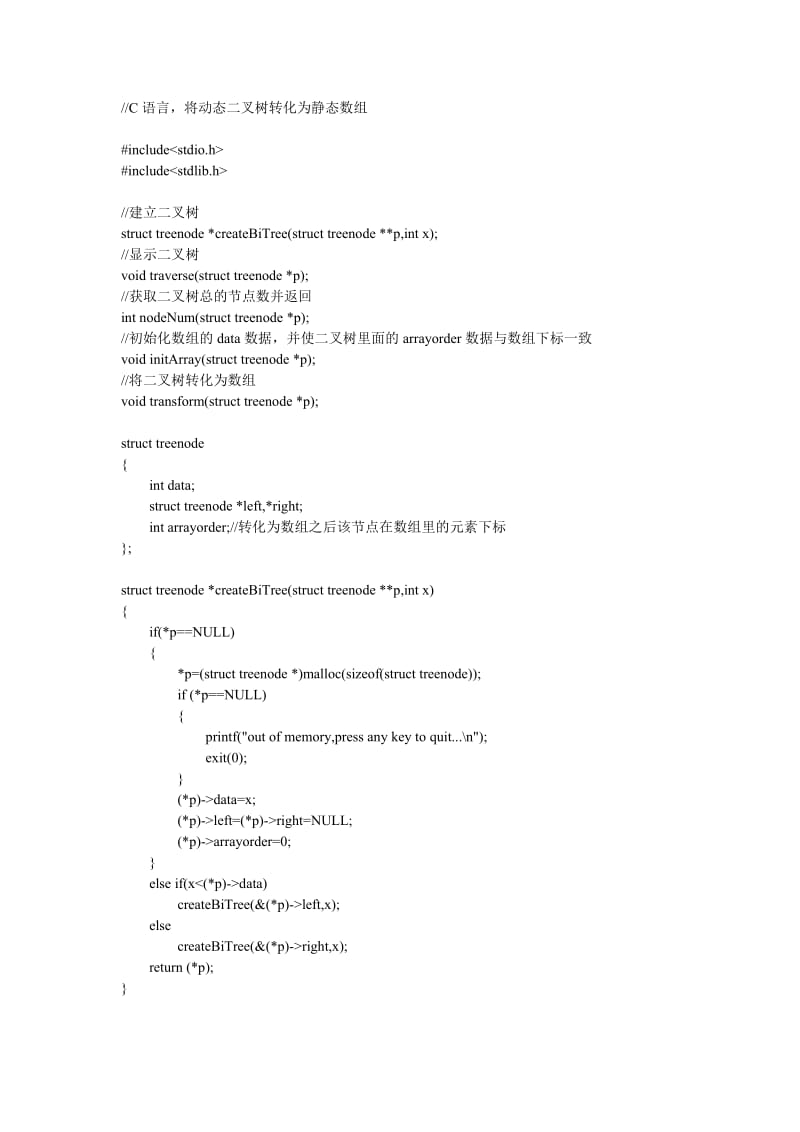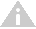• C语言 二叉树 C 数据结构 用C语言实现建立一棵二叉树 支持插入，删除结点，画出二叉树
• 按照先序顺序输入二叉树，空的位置用#表示，比如下图的就用ABD##E##C#F##表示 最后的显示效果为用括号表示二叉树的子结点，“，”前为左孩子，后面为右孩子。 下面上我拙劣的代码 #include <stdio.h> #include...
按照先序顺序输入二叉树，空的位置用#表示，比如下图的就用ABD##E##C#F##表示最后的显示效果为用括号表示的二叉树，“，”前为左孩子，后面为右孩子。 下面上我拙劣的代码(*￣︶￣)
#include <stdio.h>
#include <stdlib.h>
#include <string.h>

typedef struct Tree
{
struct Tree *lchild;
char data;
struct Tree *rchild;
} BiTree;
//创建二叉树
BiTree *CreatTree()
{
//按先序顺序输入一个二叉树，如果输入“#”，表明该二叉树为空。
char ch;
ch = getchar();
if (ch == '#')
{
return NULL;
}
else
{
BiTree *T;
T = (BiTree *)malloc(sizeof(BiTree));
T->data = ch;
T->lchild = CreatTree();
T->rchild = CreatTree();
return T;
}
}
//显示二叉树
void Show(BiTree *T)
{
if (!T)
return;
printf("%c", T->data);
if (T->lchild || T->rchild)
{
printf("(");
Show(T->lchild);

if (T->rchild)
{
printf(",");
Show(T->rchild);
}
printf(")");
}
}
int main()
{
BiTree *T = NULL;
printf("请按先序顺序输入一棵二叉树,如果输入“#”表示该位置为空：");
T = CreatTree();
Show(T);
printf("\n");
system("pause");
return 0;
}

测试用例
ABD##E##C#F## 结果：A(B(D,E),C(,F))
好啦~我的第一篇博客到这里就结束了 ~期待前辈们的指点。
展开全文数据结构
• #include #include typedef struct BiTNode { char data; struct BiTNode *lchild,*rchild; } BiTNode,*BiTree;//定义结点类型 BiTree CreateBiTree()//创建树 { char p;BiTree T; scanf("%c",&p);...}c 数据结构
• 该楼层疑似违规已被系统折叠隐藏此楼查看此楼voidCreatBiTree(BiTreeT){chara;scanf("%c",&a);if(a=='@')T=NULL;else{T=(BiTree)malloc(sizeof(BiNode));T->data=a;CreatBiTree(T->lchild);...

该楼层疑似违规已被系统折叠 隐藏此楼查看此楼
void CreatBiTree(BiTree T)
{
char a;
scanf("%c",&a);
if(a=='@')
T=NULL;
else
{
T=(BiTree)malloc(sizeof(BiNode));
T->data=a;
CreatBiTree(T->lchild);
CreatBiTree(T->rchild);
}
}
虽能编译，但不能正确运行，冥思苦想n小时，未果
遂改其为
BiTree CreatBiTree()
{
char a;
BiTree T;
scanf("%c",&a);
if(a=='@')
T=NULL;
else
{
T=(BiTree)malloc(sizeof(BiNode));
T->data=a;
T->lchild=CreatBiTree();
T->rchild=CreatBiTree();
}
return T;
}
不再用传递参数，用返回值，正确编译运行，喜。
重审前程序，改为
void CreatBiTree(BiTree *T)
{
char a;
scanf("%c",&a);
if(a=='@')
*T=NULL;
else
{
*T=(BiTree)malloc(sizeof(BiNode));
(*T)->data=a;
CreatBiTree(&((*T)->lchild));
CreatBiTree(&((*T)->rchild));
}
}
正确编译运行，惑。
又冥思苦想n小时，终于悟出其中原由(好象多难似的，或者其实是我太愚钝)
原来还是对指针的理解不够深刻
最开始的程序传递的是Node型结构体的指针，于是在函数中会生成一个形参也指向这个结构体，但在函数中有一句T=(BiTree)malloc(sizeof(BiNode)); 改变了形参的指向，以后再对形参的任何操作不会在主函数中的T产生影响。
下面是两个完整的程序，分别用的两种建树方法
#include "stdlib.h"
#include "conio.h"
typedef struct node
{
char data;
struct node *lchild,*rchild;
}BiNode,*BiTree;
BiTree CreatBiTree()
{
char a;
BiTree T;
scanf("%c",&a);
if(a=='@')
T=NULL;
else
{
T=(BiTree)malloc(sizeof(BiNode));
T->data=a;
T->lchild=CreatBiTree();
T->rchild=CreatBiTree();
}
return T;
}
void PreOrderTraverse(BiTree T)
{
if(T)
{
printf("%c",T->data);
PreOrderTraverse(T->lchild);
PreOrderTraverse(T->rchild);
}
}
void InOrderTraverse(BiTree T)
{
if(T)
{
InOrderTraverse(T->lchild);
printf("%c",T->data);
InOrderTraverse(T->rchild);
}
}
void PostOrderTraverse(BiTree T)
{
if(T)
{
PostOrderTraverse(T->lchild);
PostOrderTraverse(T->rchild);
printf("%c",T->data);
}
}
main()
{
BiTree T;
clrscr();
T=CreatBiTree();
PreOrderTraverse(T);
printf("\n");
InOrderTraverse(T);
printf("\n");
PostOrderTraverse(T);
}
二
#include "stdlib.h"
#include "conio.h"
typedef struct node
{
char data;
struct node *lchild,*rchild;
}BiNode,*BiTree;
void CreatBiTree(BiTree *T)
{
char a;
scanf("%c",&a);
if(a=='@')
*T=NULL;
else
{
*T=(BiTree)malloc(sizeof(BiNode));
(*T)->data=a;
CreatBiTree(&((*T)->lchild));
CreatBiTree(&((*T)->rchild));
}
}
void PreOrderTraverse(BiTree T)
{
if(T)
{
printf("%c",T->data);
PreOrderTraverse(T->lchild);
PreOrderTraverse(T->rchild);
}
}
void InOrderTraverse(BiTree T)
{
if(T)
{
InOrderTraverse(T->lchild);
printf("%c",T->data);
InOrderTraverse(T->rchild);
}
}
void PostOrderTraverse(BiTree T)
{
if(T)
{
PostOrderTraverse(T->lchild);
PostOrderTraverse(T->rchild);
printf("%c",T->data);
}
}
main()
{
BiTree T;
clrscr();
CreatBiTree(&T);
PreOrderTraverse(T);
printf("\n");
InOrderTraverse(T);
printf("\n");
PostOrderTraverse(T);
}
更多数据结构实例http://hi.baidu.com/longzuo

展开全文• 自己编的c语言建立二叉树的代码，运行时出错了，自己找不到错误，求大神帮忙，指点,急. #include #include typedef struct tnode Tnode; struct tnode { Tnode *left; Tnode *right; int data; }; Tnode *new_...c struct typedef 代码
• C语言 将二叉树转化为静态数组 (4页)...9.9 积分//C语言，将动态二叉树转化为静态数组#include#include//建立二叉树struct treenode *createBiTree(struct treenode **p,int x);//显示二叉树void traverse(struct tr...C语言 将二叉树转化为静态数组
(4页)本资源提供全文预览，点击全文预览即可全文预览,如果喜欢文档就下载吧，查找使用更方便哦！
9.9 积分
//C语言，将动态二叉树转化为静态数组#include#include//建立二叉树struct treenode *createBiTree(struct treenode **p,int x);//显示二叉树void traverse(struct treenode *p);//获取二叉树总的节点数并返回int nodeNum(struct treenode *p);//初始化数组的data数据，并使二叉树里面的arrayorder数据与数组下标一致void initArray(struct treenode *p);//将二叉树转化为数组void transform(struct treenode *p);struct treenode{ int data; struct treenode *left,*right; int arrayorder;//转化为数组之后该节点在数组里的元素下标};struct treenode *createBiTree(struct treenode **p,int x){ if(*p==NULL) { *p=(struct treenode *)malloc(sizeof(struct treenode)); if (*p==NULL) { printf("out of memory,press any key to quit...\n"); exit(0); } (*p)->data=x; (*p)->left=(*p)->right=NULL; (*p)->arrayorder=0; } else if(xdata) createBiTree(&(*p)->left,x); else createBiTree(&(*p)->right,x); return (*p);}static int length=0;int nodeNum(struct treenode *p){ if(p!=NULL) { length++; nodeNum(p->left); nodeNum(p->right); } return length;}void traverse(struct treenode *p){ if(p!=NULL) { printf("%d ",p->data); traverse(p->left); traverse(p->right); }}struct treeArray{ int data; int lchild,rchild;};static struct treeArray *a=NULL;//转化之后的数组static int num=0;void initArray(struct treenode *p){ if(p!=NULL) { a[num].data=p->data; p->arrayorder=num; num++; initArray(p->left); initArray(p->right); }}static int i=0;void transform(struct treenode *p){ if(p!=NULL) { if(p->left!=NULL) a[i].lchild=p->left->arrayorder; if(p->right!=NULL) a[i].rchild=p->right->arrayorder; i++; transform(p->left); transform(p->right); }}void main(void){ int x; struct treenode *root=NULL; //建立二叉树链表 printf("输入数据以\"ctrl+z\"结束:\n"); while(scanf("%d",&x)!=EOF) createBiTree(&root,x); printf("先序输出二叉树:"); traverse(root);//输出二叉链表 printf("\n"); //动态分配跟二叉树节点个数一样的静态数组 length=nodeNum(root); a=(struct treeArray *)malloc(sizeof(struct treeArray)*length); if (a==NULL) { printf("out of memory,press any key to quit...\n"); exit(0); } //用0初始化数组 for(int i=0;i天天文库所有资源均是用户自行上传分享，仅供网友学习交流，未经上传用户书面授权，请勿作他用。

展开全文• C语言编程二叉树》由会员分享，可在线阅读，更多相关《C语言编程二叉树(8页珍藏版)》请在人人文库网上搜索。1、班级： 数学101软件 学号：5姓名： 田贵文实验组别： 实验日期： 报告日期： 成绩： 报告内容：(目的...

《C语言编程二叉树》由会员分享，可在线阅读，更多相关《C语言编程二叉树(8页珍藏版)》请在人人文库网上搜索。
1、班级： 数学101软件 学号：5姓名： 田贵文实验组别： 实验日期： 报告日期： 成绩： 报告内容：(目的和要求、原理、步骤、数据、计算、小结等)实验名称：C语言编程二叉树一、实验目的及要求1.掌握二叉树的存储实现2.掌握二叉树的遍历思想3.掌握二叉树的常见算法的程序实现二、实验内容1.编写函数,输入字符序列,建立二叉树的二叉链表。2.编写函数,实现二叉树的中序递归遍历算法。(最好也能实现前缀和后缀遍历算法)3.编写函数,实现二叉树的中序非递归遍历算法。4.编写函数,借助队列实现二叉树的层次遍历算法。5.编写函数,求二叉树的高度。6.编写函数,求二叉树的结点个数。7.编写函数,求二叉树的叶子个。
2、数。8.编写函数,交换二叉树每个结点的左子树和右子树。9.编写一个主函数,在主函数中设计一个简单的菜单,分别调试上述算法。三、实验结果四、实验总结通过此实验，我掌握了二叉树的存储实现，掌握了二叉树的遍历思想，掌握了二叉树的常见算法的程序实现。附录#include #include #define MAXSIZE 100typedef char DataType;typedef struct BiTNode/* 二叉链表存储结构 */DataType data;struct BiTNode *lchild,*rchild;BiTree;typedef BiTree* ElemType ;/* 栈。
3、中数据元素类型，栈中保存结点指针 */typedef struct ElemType dataMAXSIZE;int top;SeqStack;/* 栈的类型定义，顺序栈 */typedef structElemType queueMAXSIZE;int front,rear;SP;SeqStack *initSeqStack() /* 初始化栈 */ SeqStack *s; /* 首先建立栈空间，然后初始化栈顶指针 */s=(SeqStack*)malloc(sizeof(SeqStack);s-top=-1; return s;int push(SeqStack *s,ElemType 。
4、x) if(s-top=MAXSIZE-1) /* 栈满不能入栈 */printf(栈满); return 0; s-top+;s-datas-top=x;return 1;void pop(SeqStack *s) /* 出栈，假设栈不空 */ s-top-; int empty(SeqStack *s) if(s-top=-1) return 1; else return 0; ElemType top(SeqStack *s) /* 设栈不空 */return (s-datas-top); /* 递归算法创建二叉链表 */BiTree *createBiTree()DataType ch。
5、;BiTree *T;ch=getchar();if(ch=0) return NULL;else T=(BiTree *)malloc(sizeof(BiTree);T-data=ch;T-lchild=createBiTree();T-rchild=createBiTree();return T; /* 中序遍历二叉树的递归算法 */void InOrder(BiTree *T) if(T)InOrder(T-lchild);printf(%c,T-data);InOrder(T-rchild);/* 前序遍历二叉树的递归算法 */void PreOrder(BiTree *T)if(T)。
6、printf(%c,T-data);PreOrder(T-lchild);PreOrder(T-rchild);/* 后序遍历二叉树的递归算法 */void PostOrder (BiTree *T)if(T)PostOrder(T-lchild);PostOrder(T-rchild);printf(%c,T-data);/* 中序遍历二叉树的非递归算法 */void InOrderFei(BiTree *p)SeqStack *s; s=initSeqStack();while(1)while(p) push(s,p); p=p-lchild;/* 先将结点指针压栈，待出栈时再访问 */i。
7、f(empty(s) break;p=top(s); pop(s); printf(%c,p-data); p=p-rchild;/* 按层次遍历 */void LevelOrder(BiTree *T) SP *p;p=(SP*)malloc(sizeof(SP);p-front=0;p-rear=0;if(T!=NULL)p-queuep-front=T;p-front=p-front+1;while(p-front!=p-rear)T=p-queuep-rear; p-rear=p-rear+1;printf(%c,T-data);if(T-lchild!=NULL)p-queuep-f。
8、ront=T-lchild;/*左孩子进队列*/p-front=p-front+1;if(T-rchild!=NULL)p-queuep-front=T-rchild;/*右孩子进队列*/p-front=p-front+1; /* 求二叉树的高度 */int height(BiTree *T)int i,j;if(!T) return 0;i=height(T-lchild);/* 求左子树的高度 */j=height(T-rchild);/* 求右子树的高度 */return ij?i+1:j+1;/* 二叉树的高度为左右子树中较高的高度加1 */* 求二叉树的所有结点个数 */int No。
9、des(BiTree *T) int n1,n2;if(T=NULL) return 0;else if(T-lchild=NULL&T-rchild=NULL) return 1;else n1=Nodes(T-lchild);n2=Nodes(T-rchild);return n1+n2+1; /* 求二叉树的叶子结点个数 */int leafs(BiTree *T)int num1,num2;if(T=NULL) return 0;elseif(T-lchild=NULL&T-rchild=NULL) return 1;num1=leafs(T-lchild);/* 求左子树中叶子结点数。
10、 */num2=leafs(T-rchild);/* 求右子树中叶子结点数 */return num1+num2; /* 交换二叉树的所有左右子树 */void exchange(BiTree *T) BiTree *temp=NULL;if(T-lchild=NULL&T-rchild=NULL) return;elsetemp=T-lchild;T-lchild=T-rchild;T-rchild=temp;if(T-lchild) exchange(T-lchild);if(T-rchild) exchange(T-rchild);/* 交换后二叉树的遍历 */void Display(。
12、.层次-遍历二叉树n);printf(tt7.二叉树的高度n);printf(tt8.二叉树的结点个数n);printf(tt9.二叉树的叶子结点个数n);printf(tt10.交换二叉树的所有左右子树n);printf(tt0.退出系统n);printf(nt请选择:); void main() BiTree *bt; bt=NULL;int n,m=1;while(m)menu(); scanf(%d,&n); getchar();switch(n)case 1:printf(nt请输入结点的前序序列创建二叉树:0表示空:);bt=createBiTree(); break;/* 生成二。
13、叉树 */case 2:printf(nt递归-中序遍历二叉树:);InOrder(bt);printf(n); break;case 3:printf(nt递归-前序遍历二叉树:);PreOrder(bt); printf(n); break;case 4:printf(nt递归-后序遍历二叉树:);PostOrder(bt);printf(n); break;case 5:printf(nt非递归-中序遍历二叉树);InOrderFei(bt);printf(n); break;case 6:printf(nt按层次遍历二叉树:);LevelOrder(bt);printf(n); break;case 7:printf(nt二叉树的高度为:%dn,height(bt);printf(n); break;case 8:printf(nt二叉树的结点数为:%dn,Nodes(bt);printf(n); break;case 9:printf(nt二叉树中叶子结点数为:%dn,leafs(bt); break;case 10:printf(nt交换二叉树的所有左右子树);printf(nn);exchange(bt);Display(bt); break;case 0:m=0;。

展开全文• C语言】二叉树（按层建立二叉树，前中后序以及按层遍历） 二叉树建立的方式有很多，最常见的是我们看到的递归建立从左到右的方法，这边分享一种要求逐层建立二叉树的题目 问题：A binary tree can be uniquely ...代码 算法
• C 语言编程二叉树实验名称：C语言编程二叉树一、实验目的及要求1.掌握二叉树的存储实现2.掌握二叉树的遍历思想3.掌握二叉树的常见算法的程序实现二、实验内容1.编写函数 ,输入字符序列 ,建立 二叉树的 二叉链表 。2....
• C语言实现二叉树的创建以及遍历#include typedef char ElemType;typedef struct BiTNode{ElemType data;struct BiTNode *lchild;...//先序建立二叉树方法一void CreateBiTreeMethod1(BiTree *T);//先序建...
• 详细的讲解了二叉树用C语音的建立过程。并实现了相应的遍历。删除功能。。
• C语言_二叉树建立、遍历、交换子树代码、是可执行的源代码，欢迎大家下载
• 二叉树的定义：二叉树由节点...按照先序序列输入的数据构建一棵由先序方式构建的二叉树：代码以下/* 先序建立一棵任意二叉树 *//* 注意：输入数据的顺序颇有特色，本题输入的顺序要求为，先是根节点，再是左子树，再...
• 然后，便是对已经建立二叉树进行线索化。 上图是对头结点Thrt的初始化，结点pre为全局变量。 另外需要记住： （1）LTag为0时指向该结点的左孩子，为1时指向该结点的前驱； （2）RTag为0时指向该结点的右孩子，为1...数据结构 链表 指针
• #include <bits/stdc++.h> using namespace std; typedef struct treenode { char val;//结点 struct treenode *left;//建立左指针 struct treenode *right;...t)//建立二叉树 { char ch; cin>>.数据结构
• C语言实现二叉树的各种遍历及求解深度 一、介绍 二叉树是一种重要的数据结构，在很多方面都有重要的应用，此文主要记录了二叉树的基础知识，包括二叉树建立、前中后序遍历方式、层次遍历方式、求解二叉树的深度...层次遍历 后序遍历
• ## C语言创建二叉树

千次阅读 2018-10-05 15:46:02
//C语言实现创建二叉树 #include&lt;stdio.h&gt; #include&lt;stdlib.h&gt; typedef int DataType; typedef struct Node{ DataType Data; struct Node *lchild, *rchild; }BinTNode, *BiTree;...
• C语言实现的二叉搜索树，部分功能如下： 1.树的建立、清空、删除 2.先序、中序、后序的递归和非递归输出 3.插入 4.寻找数，寻找最大最小值 5.返回树高度
• (2)掌握二叉树的链式存储结构的建立算法 (3 掌握二叉表上二叉树的基本运算的实现 二：实验内容 （1）用递归实现二叉树的前序，中序，后序的3种遍历 （2）用非递归实现二叉树的前序，中序，后序3种遍历 三：实验...数据结构
• 通过前序建立二叉树时，会有很多个空指针存在 对于拥有N个结点的链表，拥有N+1个空指针域，即是空指针。 只有通过遍历，产生一个序列，才知道一个结点的前驱和后继（线性表），否者只能知道他的左右子树 利用空...
• 一、介绍二叉树是一种重要的数据结构，在很多方面都有重要的应用，此文主要记录了二叉树的基础知识，包括二叉树建立、前中后序遍历方式、层次遍历方式、求解二叉树的深度、求解二叉树的节点总数、求解二叉树每层的...
• 建立时按先序输入的结点序列为：abc###de#f##g##（2）基本要求每个运算要求用一个函数实现。主要运算为：创建二叉树二叉树的先序遍历、二叉树的中序遍历、二叉树的后序遍历。（3）算法描述 参考教材算法6.4。（4...
• C语言二叉树的中序遍历标签： C语言 二叉树 中序遍历by 小威威这周作业题目是关于二叉树的中序遍历。中序遍历就是先读取左子树，再读取根结点，最后再读取右子树。在本题中的意思就是先输出左子树，再输出根结点，...遍历
• 第一行 为自己输入的数据，在创建的二叉树中数据域为char型，故空格和enter键也会被存入二叉树数据中。‘#’号总比二叉树数据结点多一个，不然一直在输入，无法进入输出。输出是中序遍历打印的。 根据上面的输入...数据结构 链表 指针
• ## C语言实现二叉树的非递归遍历

千次阅读 多人点赞 2018-04-26 21:29:56
C语言实现二叉树的非递归遍历： 代码解释：非递归前序遍历：  1&gt; 首先建立一个二维指针，用来存储每个结点的地址，定义栈顶指针top，初始值为-1，并将根结点存入栈中，top++；  2&gt; 进入while循环，...
• C语言实现二叉树的分层遍历：代码难点：  第一点：先将初始化过得数组，变成符合规范的二叉树(规范：双亲结点大于左子树，小于右子树)的过程；  第二点：存储结点的队列，只要输出一个结点，就将它的左右子树按照...
• 编写函数，输入字符序列，建立二叉树的二叉链表。2. 编写函数，实现二叉树的中序递归遍历算法。(最好也能实现前缀和后缀 遍历算法)3. 编写函数，实现二叉树的中序非递归遍历算法。4. 编写函数，借助队列实现二叉树......

# c语言建立二叉树c语言 订阅